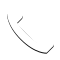Select Page

Loan refinancing occurs with buyers who are currently paying an interest rate which is more than the current market rate. This usually occurs when interest rates are low. Refinancing is done by taking a new loan from another bank at a lower interest rate. While using the new loan to repay all the remaining balance of the existing loan to the original bank. Simply put, you are changing a bank for your mortgage loan.

### Things to Consider For Refinancing

• Terms of the current outstanding loan
• What are the new loan terms?
• Fees associated with the whole refinancing process?
• Calculating the ‘breakeven’ interest rate so that refinancing is suitable for me?

### Break-even Interest Rate

Example:

• 6 years ago, you borrowed \$225,000 to purchase a home with a 7.5%, 25-year, fixed-rate mortgage, with the lock-in period of 10 years (2% prepayment penalty for the first 10 years)
• You will live in the house for 7 more years
• You have the option to refinance the house into a new 6.0%, 25-year, fixed-rate mortgage with 3% fees, with the 5-year lock-in (No prepayment penalty after 5 years)

How to start?

1. Firstly, we want to calculate the amount of monthly mortgage payment, remaining balance so we know how much we need to borrow. Remember that the amount we want to borrow must be able to also pay off our cost/service fees for the refinance.
2. We want to find out the total cost involved(the amount to be loaned) by changing to another bank
3. We also find out how much loan balance is left if you continue with the current loan for another 7 years.
4. Using the information calculate the ‘break-even’ interest rate.

Calculate Monthly Payment

• P/Y =12
• N = 25*12 = 300
• I/Y = 7.5%
• PV = \$225,000
• FV=0
• CPT PMT=-\$1662.73

Remaining Loan Balance After 6 Years

• AMORT : P1=1, P2=6*12=72
• BAL = \$201,768.34

Total Amount Of New Loan Needed

• Payment for prepayment penalty: \$201,768.34(1.02) = \$205,803.71
• Payment fees for new mortgage: \$205,803.71(1.03) = \$211,977,82 (Final Amount Needed)

Remaining Loan Balance After 13 Years With Current Loan

• AMORT: P1=1, P2=(6+7)*12=156
• BAL = \$157,570.95

Calculate Break-even Interest Rate For 7 Years (Assuming With Original Loan)

• P/Y = 12
• N = 7*12 = 84
• PV = \$211,977.81 (value of the total loan needed)
• FV = -\$157,570.94 (total loan repayment after 7 years)
• PMT = -\$1662.73 (monthly payment)
• CPT I/Y = 6.51%

It would be easier to think it this way. If you have kept the previous loan while adding the cost(refinance cost, prepayment penalty and mortgage fees) to your current loan. What is the actual interest rate to breakeven the increase in loan amount? Because if you think about it, your loan amount increases but your monthly payment and ending loan balance stays the same, it would mean that the interest rate is lower. This lowered interest rate is the break-even interest rate as you want, to stay indifferent between the first and second loan.

##### What Does ‘Break-even’ Interest Rate Mean?

The Break-even interest rate is a rate that you need to have in order to be indifferent to the current loan. Therefore, if current interest rates are lower than the Break-even interest rate it is beneficial to refinance. If the current interest rate is higher than the Break-even interest rate, you should not refinance.

## Get In Touch

5 + 14 =Click To Call